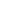top
The tensile strength of thick-walled stainless steel pipe is too strong1 Thick-walled stainless steel pipes have good tensile strength, tensile strength and sufficient toughness, which makes thick-walled stainless steel pipes stand out in the field of steel pipes and are brilliant. Thick-walled stainless steel pipes have great advantages in high temperature and high pressure and even in high-strength environments. What are their characteristics? We analyze one by one; the tensile strength (φb) sample is in the stretching process Among them, the maximum force (Fb) that is borne when it is broken is the stress (o) obtained from the original cross-sectional area (So) of the sample, which is called the tensile strength (φb), and the unit is N/mm2 (MPa) .

2.It represents the maximum capacity of metal materials to resist damage under tensile force. The calculation formula is: where: Fb-the maximum force that the specimen bears when it is broken, Ñ (Newton; So-the original cross-sectional area of ​​the specimen, mm2. , The stress at which Xiangxiang can continue to elong without increasing the force during the stretching process (remaining constant) is called the yield point.

3.If the force drops, the upper and lower yield points should be distinguished. The unit of yield point is N/mm2 (MPa). Upper yield point (φsu): the maximum stress before the sample yields and the force drops for the first time; lower yield point (os): the minimum stress in the yield section when the initial transient effect is not taken into account. The calculation formula of the yield point is: where: Fs-yield force during the tensile process of the sample (constant), N (Newton) So-the original cross-sectional area of ​​the sample, mm2. Elongation after break (φ) In a tensile test, the percentage of the length of the gauge length increased by the specimen after it is broken to the original gauge length is called the elongation

4.Expressed by φ, the unit is %. The calculation formula is: In the formula: L1-the gauge length after the sample is broken, mm; L0-the original gauge length of the sample, mm. Reduction of area (ψ) In a tensile test, the percentage of the maximum reduction of the cross-sectional area at the reduced diameter of the sample after the sample is broken to the original cross-sectional area is called the reduction of area. It is expressed in %. The calculation formula is as follows : Where: S0- the original cross-sectional area of ​​the sample, mm2; 1- the minimum cross-sectional area at the reduced diameter of the sample after it is broken, mm2.Chat with Vincent PorterChat with Vincent PorterChat with Vincent PorterChat with Vincent PorterChat with Vincent Porter